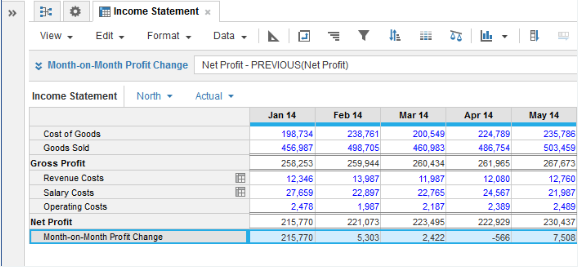1. Calculation functions
2. All Functions
3. Time and Date Functions
4. PREVIOUSReturns the value from the previous period.

## Syntax

PREVIOUS(x)

where:

• x: Line item: Number, Boolean, date, time period, list item, text

## Format

Input Format Output Format

Number, Boolean, date, time period, list item, text

Matches the data format used by argument x

## Arguments

The function uses the following arguments:

• x: Number, Boolean, date, time period, list item, text

## Constraints

The function has the following constraints:

• The result formatting must match the source formatting.
• If the source is list-formatted, then the result must be formatted to the same list.
• If the source is time period-formatted, then the result must be formatted for the same time period. For example, if the source is time period-formatted as Month, then the result must also be formatted as Month.

## Excel equivalent

• No Excel equivalent

## Example

In this example, we have a simple Income Statement module, with line items on rows, a time dimension on columns, and Organization and Versions as pages. The number-formatted Net Profit line item shows calculated net profit each month and is summed for quarters. We can use the PREVIOUS function in the number-formatted Month-on-Month Profit Change result line item against the Net Profit source to track profit changes for the actual version:

Month-on-Month Profit Change = Net Profit - PREVIOUS(Net Profit)